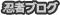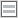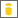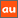# 0除算に関するアナウンスメントを公開しています

The purpose of this site is to publish announcements on the theory of division by zero

## Announcement 409: Various Publication Projects on the Division by Zero

Institute of Reproducing Kernels
Kawauchi-cho, 5-1648-16,
Kiryu 376-0041, Japan
January 29, 2018

The Institute of Reproducing Kernels is dealing with the theory of division by zero calculus and declares that the division by zero was discovered as 0/0 =1/0 = z/0 = 0 in a natural sense on 2014.2.2. The result shows a new basic idea on the universe and space since Aristoteles $($BC384 - BC322$)$ and Euclid $($BC 3 Century - $)$, and the division by zero is since Brahmagupta $($598 - 668?$)$. In particular, Brahmagupta defined as $0/0 = 0$ in Brhmasphuasiddhnta $($628$)$, however, our world history stated that his definition $0/0 = 0$ is wrong over 1300 years, but, we showed that his definition is suitable. For the details, see the references and the site: http://okmr.yamatoblog.net/
We wrote two global book manuscripts  with 154 pages and  with many figures for some general people. Their main points are:

• The division by zero and division by zero calculus are new elementary and fundamental mathematics in the undergraduate level.

• They introduce a new space since Aristoteles $($BC384 - BC322$)$ and Euclid $($BC 3 Century - $)$ with many exciting new phenomena and properties with general interest, not specialized and difficult topics. However, their properties are mysterious and very attractive.

Meanwhile, the representations of the contents are very important and delicate with delicate feelings to the division by zero with a long and mysterious history. Therefore, we hope the representations of the division by zero as follows:

• Various book publications by many native languages and with the author’s idea and feelings.

• Some publications are like arts and some comic style books with pictures.

• Some T shirts design, some pictures, monument design may be consided.

The authors above may be expected to contribute to our culture, education, common communications and enjoyments. For the people having the interest on the above projects, we will send our book sources with many figure files.
How will be our project introducing our new world since Euclid?
Of course, as mathematicians we have to publish new books on Calculus, Differential Equations and Complex Analysis, at least and soon, in order to correct them in some complete and beautiful ways.
Our topics will be interested in over 1000 millions people over the world on the world history.

References
 M. Kuroda, H. Michiwaki, S. Saitoh, and M. Yamane, New meanings of the division by zero and interpretations on 100/0 = 0 and on 0/0 = 0, Int. J. Appl. Math. 27 $($2014$)$, no 2, pp. 191-198, DOI:
10.12732/ijam.v27i2.9.
 T. Matsuura and S. Saitoh, Matrices and division by zero $z/0 = 0$, Advances in Linear Algebra & Matrix Theory, 6$($2016$)$, 51-58 Published Online June 2016 in SciRes. http://www.scirp.org/journal/alamt
 T. Matsuura and S. Saitoh, Division by zero calculus and singular integrals. $($Submitted for publication$)$
 T. Matsuura, H. Michiwaki and S. Saitoh, log0 = log∞ = 0 and applications. Differential and Difference Equations with Applications.Springer Proceedings in Mathematics & Statistics.
 H. Michiwaki, S. Saitoh and M.Yamada, Reality of the division by zero z/0 = 0. IJAPM International J. of Applied Physics and Math. 6$($2015$)$, 1–8. http://www.ijapm.org/show-63-504-1.html
 H. Michiwaki, H. Okumura and S. Saitoh, Division by Zero z/0 = 0 in Euclidean Spaces, International Journal of Mathematics and Computation, 28$($1$)$$($2017$)$; 1-16.
 H. Okumura, S. Saitoh and T. Matsuura, Relations of 0 and ∞, Journal of Technology and Social Science $($JTSS$)$, 1$($2017$)$, 70-77.
 H. Okumura and S. Saitoh, The Descartes circles theorem and division by zero calculus. https://arxiv.org/abs/1711.04961 $($2017.11.14$)$.
 H. Okumura, Wasan geometry with the division by 0. https://arxiv.org/abs/1711.06947 International Journal of Geometry.
 H. Okumura and S. Saitoh, Applications of the division by zero calculus to Wasan geometry. $($Submitted for publication$)$.
 S. Pinelas and S. Saitoh, Division by zero calculus and differential equations. Differential and Difference Equations with Applications. Springer Proceedings in Mathematics & Statistics.
 H. G. Romig, Discussions: Early History of Division by Zero, American Mathematical Monthly, Vol. 31, No. 8.$($Oct., 1924$)$, pp. 387-389.
 S. Saitoh, Generalized inversions of Hadamard and tensor products for matrices, Advances in Linear Algebra & Matrix Theory. 4 $($2014$)$, no. 2, 87–95. http://www.scirp.org/journal/ALAMT/
 S. Saitoh, A reproducing kernel theory with some general applications, Qian,T./Rodino,L.(eds.): Mathematical Analysis, Probability and Applications - Plenary Lectures: Isaac 2015, Macau, China, Springer Proceedings in Mathematics and Statistics, 177$($2016$)$, 151-182. $($Springer$)$.
 S. Saitoh, Mysterious Properties of the Point at Infinity, arXiv:1712.09467 [math.GM]$($2017.12.17$)$.
 S. Saitoh, Division by zero calculus $($154 pages: draft$)$: http//okmr.yamatoblog.net/
 S. Saitoh and H. Okumura, Division by Zero Calculus in Figures – our New Space –
 S.-E. Takahasi, M. Tsukada and Y. Kobayashi, Classification of continuous fractional binary operations on the real and complex fields, Tokyo Journal of Mathematics, 38$($2015$)$, no. 2, 369-380.
PR

### コメント

お名前 Black LimeGreen SeaGreen Teal FireBrick Tomato IndianRed BurlyWood SlateGray DarkSlateBlue LightPink DeepPink DarkOrange Gold DimGray Silverプロフィール

ＨＮ：